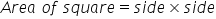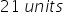Mathematics
Easy

Question

# What is the length of the side of a square, if the area of the square is 441 cm2

## 21 cm29 cm31 cm39 cmHint:

## The correct answer is: 21 cm

### Here we have given a square of area 441 square centimeters and we have to depict the side length of that square. The quantity of square units required to completely fill a square is known as the area of a square. Measurements are made in square units, with square meters.We will use the concept of square root to find the sides of the square. For this, we need to know the area of square, which is:Now we have given the area, so lets put it in the formula, we get:So the side of the square is.

In this question, we have given the area of the square and we have to find the side length of the square. So we used the concept of a square function and using that we first found the side of the square which was found to be 21 centimeters.

### Related Questions to study#### With Turito Foundation.#### Get an Expert Advice From Turito.